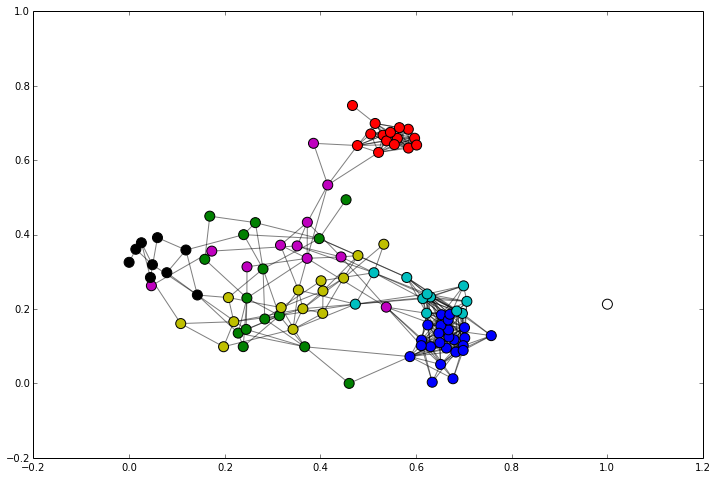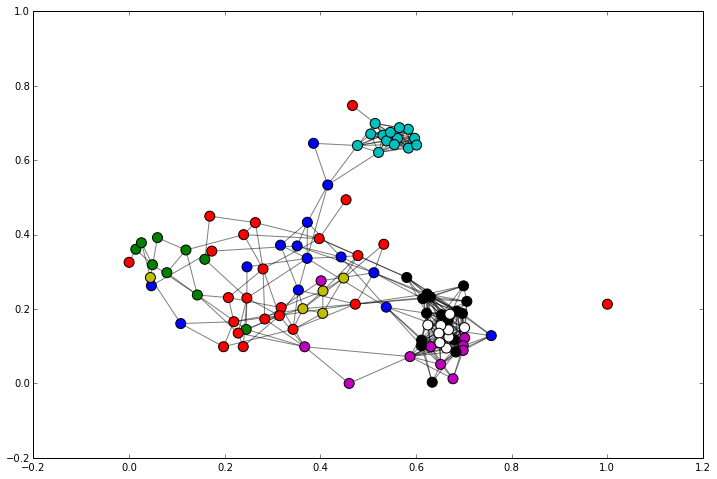# Autotelic Computing

## Thursday, March 20, 2014

### K-means vs Louvain

I'm currently reading Data Smart, a particularly good and entertaining book about machine learning and data science. The somewhat surprising twist in its approach is that it does almost entirely without code, and instead implements and illustrates everything in terms of spreadsheet operations. This has produced an interesting contradiction in me: the coding purist is somewhat put off by what he always perceived as a clunky and underpowered computing paradigm, while the pragmatist is intrigued by the possible productivity gains that might result from taking the time to learn properly about such an ubiquitous tool (which I'll admit I never did). That said, the author does an excellent job at teaching complex algorithms with (not so simple) spreadsheets, which after a while almost feels like a "declarative" way of modeling problems, a refeshing take I think for people used to a more procedural way of thinking.

At some point though the use of a solver becomes inevitable for such problems (which almost always imply an optimization component), and this is another aspect of the book that I found surprisingly enlightening. Instead of delving in the intricacies of particular algorithms, it provides a unified and abstract methodology, useful to solve a large class of problems, and allows to get a deeper feel of the way they're related. The price there is to pay is that using an embedded solver can often be less efficient than a specialized algorithm, and in my case, since I only have access to LibreOffice, the pain is particularly acute for certain problems.

In the second chapter of the book, we learn about $k$-means clustering using a toy dataset in which we have the selections made by 100 clients among 32 distinct wine deals. The clients get clustered in the 32-dimension space spanned by their wine tastes, which is easy to understand in terms of abstract geometry, but rather hard to visualize. Cosine similarity is introduced as a metric making more sense and yielding better results than Euclidean distance in this particular context. Then a few chapters later, the same problem is revisited, but this time from the perspective of graph theory, from which a very clever clustering method has been devised, based on the concept of modularity (clustering in this context is rather called community detection). A graph is first constructed from the cosine similarity matrix, which can be literally interpreted as an adjacency matrix. We then cluster the nodes of this graph according to whether they share an edge or not, but with the adjustment that highly probable connections are less important, and vice versa. This can be solved with an algorithm called the Louvain method.

Since I decided to follow along using Python, I thought it would be nice to use the graph visualization to compare the results of $k$-means clustering against those of modularity maximization. Using a couple of very powerful Python libraries (Numpy, Scikit-Learn, NetworkX and Matplotlib), this is really easy. Let's first download and extract the data directly from the source:

In :
import numpy as np
import networkx as nx
import matplotlib.pyplot as plt
from sklearn.metrics.pairwise import cosine_similarity
from sklearn.cluster import KMeans
import urllib, zipfile, StringIO, xlrd, community

# unzip it
# extract the Excel file
matrix_sheet = x.sheet_by_name('Matrix')


Next let's read its content to feed a $32 \times 100$ numpy matrix, which we then transpose to obtain a dataset of customers and their wine preferences:

In :
# input data
D = np.zeros((32, 100))

for i in range(1, 33):
for j in range(7, 107):
v = matrix_sheet.cell_value(i, j)
if v: D[i-1][j-7] = 1

D = D.T # 100 x 32
D

Out:
array([[ 0.,  0.,  0., ...,  1.,  0.,  0.],
[ 0.,  0.,  0., ...,  0.,  0.,  0.],
[ 0.,  0.,  0., ...,  0.,  0.,  0.],
...,
[ 1.,  0.,  0., ...,  0.,  1.,  0.],
[ 0.,  0.,  0., ...,  0.,  0.,  0.],
[ 0.,  0.,  0., ...,  0.,  1.,  1.]])


We compute the cosine similarities of the clients, which will become an adjacency matrix, after having been constrained by the $r$-neighborhood (with $r=0.5$):

In :
S = cosine_similarity(D) # 100 x 100
S[S <= 0.49999] = 0 # using < 0.5 is not working well here for some reason
S[S != 0] = 1
S

Out:
array([[ 1.,  0.,  0., ...,  0.,  0.,  0.],
[ 0.,  1.,  0., ...,  0.,  0.,  0.],
[ 0.,  0.,  1., ...,  0.,  0.,  0.],
...,
[ 0.,  0.,  0., ...,  1.,  0.,  0.],
[ 0.,  0.,  0., ...,  0.,  1.,  0.],
[ 0.,  0.,  0., ...,  0.,  0.,  1.]])


We construct the graph from this matrix, and apply community detection on it:

In :
# S as adjacency matrix
G = nx.from_numpy_matrix(S)

# Louvain method for community detection
partition = community.best_partition(G)


We can draw the results:

In :
rcParams['figure.figsize'] = 12, 8

pos = nx.spring_layout(G, k=0.05)
colors = 'bgrcmykw'
for i, com in enumerate(set(partition.values())):
list_nodes = [nodes for nodes in partition.keys()
if partition[nodes] == com]
nx.draw_networkx_nodes(G, pos, list_nodes,
node_size=100, node_color=colors[i])

nx.draw_networkx_edges(G, pos, alpha=0.5);Finally we have a way to visualize and compare the results obtained with $k$-means:

In :
k = len(set(partition.values())) # use number of clusters found by MM (8)
kmeans = KMeans(k)
kmeans.fit(D)

for i in range(k):
list_nodes = np.where(kmeans.labels_ == i).tolist()
nx.draw_networkx_nodes(G, pos, list_nodes,
node_size=100, node_color=colors[i])
nx.draw_networkx_edges(G, pos, alpha=0.5);